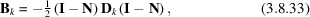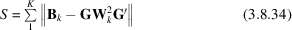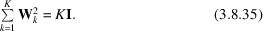International
Tables for
Crystallography
Volume H
Powder diffraction
Edited by C. J. Gilmore, J. A. Kaduk and H. Schenk

International Tables for Crystallography (2018). Vol. H, ch. 3.8, pp. 340-342

## Section 3.8.9. Combining data types: the INDSCAL method

C. J. Gilmore,a G. Barra and W. Donga*

aDepartment of Chemistry, University of Glasgow, University Avenue, Glasgow, G12 8QQ, UK
Correspondence e-mail:  chris@chem.gla.ac.uk

### 3.8.9. Combining data types: the INDSCAL method

| top | pdf |

It is now common to collect more than one data type, and some instruments now exist for collecting spectroscopic and PXRD data on the same samples, for example the Bruker D8 Screenlab, which combines PXRD and Raman measurement for high-throughput screening (Boccaleri et al., 2007).

A technique for combining the results of more than one data type is needed. One method would be to take individual distance matrices from each data type and generate an average distance matrix using equation (3.8.3), but this leaves open the question of how best to define the associated weights in an optimal, objective way. Should, for example, PXRD be given a higher weight than Raman data? The individual differences scaling method (INDSCAL) of Carroll & Chang (1970) provides an unbiased solution to this problem by, as the name suggests, scaling the differences between individual distance matrices.

In this method, let Dk be the squared distance matrix of dimension (n × n) for data type k with a total of K data types. For example, if we have PXRD, Raman and differential scanning calorimetry (DSC) data for each of n samples, then K = 3. A group-average matrix G (which we will specify in two dimensions) is required that best represents the combination of the K data types. To do this, the D matrices are first put into inner-product form by the double-centring operation to givewhere I is the identity matrix and N is the centring matrix I11′/N; 1 is a column vector of ones. The inner-product matrices thus generated are matched to the weighted form of the group average, G, which is unknown. To do this the functionis minimized. The weight matrices, Wk, are scaled such thatThe INDSCAL method employs an iterative technique to solve equation (3.8.7)in which one parameter is kept fixed whilst the other is determined by least-squares refinement. An initial estimate for G is taken either from the average of the D matrices for each sample or as a random matrix. This is then used to estimate the weight matrices, and the whole process repeated until a minimum value of S is obtained. The algorithm derived by Carroll and Chang was used in the example below. When random matrices are used to generate the initial G matrix, the INDSCAL procedure is repeated 100 times and the solution with the minimum value of S is kept. In practice, there is very little difference in the results of these two procedures. The resulting G matrix is used as a standard-distance matrix, and used in the standard way to generate dendrograms, MMDS plots etc. The method has the property that where data types show samples to be very similar this is reinforced, whereas where there are considerable variations the differences are accentuated in the final G matrix. For a fuller description of the INDSCAL method with examples see Gower & Dijksterhuis (2004), Section 13.2, and for a useful geometric interpretation see Husson & Pagès (2006).

#### 3.8.9.1. An example combining PXRD and Raman data

| top | pdf |

We now present an example of the INDSCAL method applied to data collected on sulfathiazole using PXRD and Raman spectroscopy (Barr, Cunningham et al., 2009). A flowchart is shown in Fig. 3.8.15. Three polymorphs of sulfathiazole were prepared and PXRD data were collected on a Bruker C2 GADDS system. Each sample was run for 2 min over a 3–30° range in 2θ using Cu Kα radiation. Raman data were collected on a Bruker SENTINEL. The Raman probe was integrated into the PXRD instrument.Figure 3.8.15 | top | pdf |A flowchart for the INDSCAL method using Raman and PXRD data. Note that any combination of any 1D data can be used here.

The only data pre-processing performed was background removal. Fig. 3.8.16(a) shows the resulting dendrogram (with the default cut level) and Fig. 3.8.16(b) shows the corresponding MMDS plot. To identify each sample they are numbered via a four-digit code: the first two digits are the well number, and the last digit defines whether the sample is form 2, 3 or 4 of sulfathiazole. It can be seen that the clustering is only partly successful: form 4 (red) is correctly clustered; form 3 (orange) gives five clusters and form 2 gives three clusters.Figure 3.8.16 | top | pdf |Clustering 48 PXRD spectra with background corrections applied for three polymorphs of sulfathiazole. (a) The dendrogram. Each sample is identified by a four-digit code. The first two digits are the well number, and the last digit defines whether the sample is form 2, 3 or 4 of sulfathiazole. (b) The MMDS plot: the red cluster is well defined but the rest of the spheres are diffuse and intermingled. (c) The dendrogram derived from clustering 48 Raman spectra of sulfathiazole with background corrections applied. (d) The corresponding MMDS plot. The clusters are poorly defined. (e) The results of the INDSCAL method. The dendrogram is shown with the default cut level. The clustering is correct; all the samples are placed in the correct group except for patterns 35-2 and 45-2. (f) The MMDS plot validates the dendrogram. (g) The Raman patterns for 35-2 and 45-2 superimposed. They are primarily background noise.

Fig. 3.8.16(c) shows the clustering from the Raman spectra. The results are poor: most of form 2 is correctly clustered, but forms 4 and 3 are intermixed, and the MMDS plot in Fig. 3.8.16(d) is diffuse with little structure.

The INDSCAL method is now applied starting from random G matrices and the results are shown in Fig. 3.8.16(e) and (f) with the dendrogram cut level at its default value. The clustering is almost correct; all the samples are placed in the correct groups except that there are two outliers coloured in blue. Fig. 3.8.16(g) shows the Raman patterns for these samples: they are primarily background with very little usable signal.

### References

Barr, G., Cunningham, G., Dong, W., Gilmore, C. J. & Kojima, T. (2009). High-throughput powder diffraction V: the use of Raman spectroscopy with and without X-ray powder diffraction data. J. Appl. Cryst. 42, 706–714.Google Scholar
Boccaleri, E., Carniato, F., Croce, G., Viterbo, D., van Beek, W., Emerich, H. & Milanesio, M. (2007). In situ simultaneous Raman/high-resolution X-ray powder diffraction study of transformations occurring in materials at non-ambient conditions. J. Appl. Cryst. 40, 684–693.Google Scholar
Carroll, J. D. & Chang, J. J. (1970). Analysis of individual differences in multidimensional scaling via n-way generalization of `Eckhart–Young' decomposition. Psychometria, 35, 283–319.Google Scholar
Gower, J. C. & Dijksterhuis, G. B. (2004). Procrustes Problems. Oxford University Press.Google Scholar
Husson, F. & Pagès, J. (2006). INDSCAL model: geometrical interpretation and methodology. Comput. Stat. Data Anal. 50, 358–378.Google Scholar